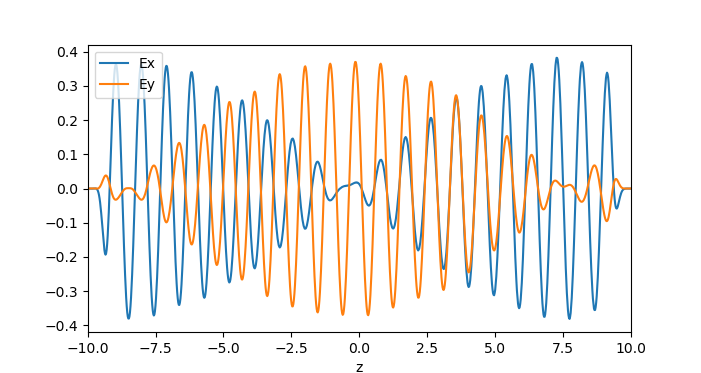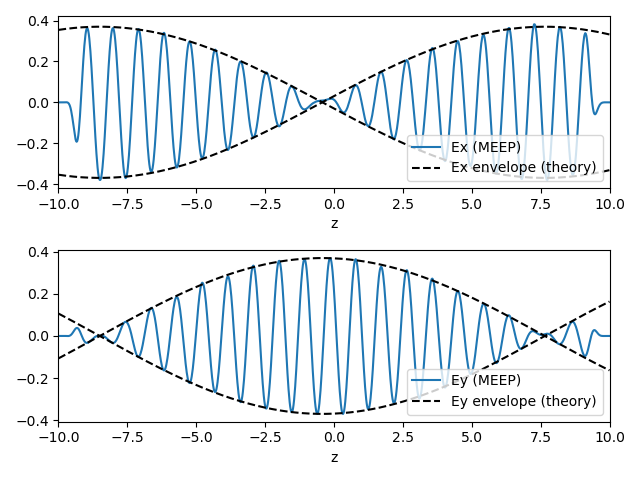# Gyrotropic Media

In this example, we will perform simulations with gyrotropic media. See Materials for more information on how gyrotropy is supported.

Consider a uniform gyroelectric medium with bias vector $\mathbf{b} = b \hat{z}$. In the frequency domain, the x and y components of the dielectric tensor have the form

The skew-symmetric off-diagonal components give rise to Faraday rotation: when a plane wave linearly polarized along x is launched along the gyrotropy axis z, the polarization vector will precess around the gyrotropy axis as the wave propagates. This is the principle behind Faraday rotators, devices that act as one-way valves for light.

A plane wave undergoing Faraday rotation can be described by the complex ansatz

where $\kappa_c$ is the Faraday rotation (in radians) per unit of propagation distance. Substituting this into the frequency domain Maxwell's equations, with the above dielectric tensor, yields

We model this phenomenon in the simulation script faraday-rotation.py. First, we define a gyroelectric material:

import meep as mp

## Parameters for a gyrotropic Lorentzian medium
epsn = 1.5    # background permittivity
f0   = 1.0    # natural frequency
gamm = 1e-6   # damping rate
sn   = 0.1    # sigma parameter
b0   = 0.15   # magnitude of bias vector

susc = [mp.GyrotropicLorentzianSusceptibility(frequency=f0, gamma=gamma, sigma=sigma,
bias=mp.Vector3(0, 0, b0))]
mat = mp.Medium(epsilon=epsn, mu=1, E_susceptibilities=susc)


The GyrotropicLorentzianSusceptibility object has a bias argument that takes a Vector3 specifying the gyrotropy vector. In this case, the vector points along z, and its magnitude (which specifies the precession frequency) is determined by the variable b0. The other arguments play the same role as in an ordinary (non-gyrotropic) Lorentzian susceptibility.

Next, we set up and run the Meep simulation.

tmax = 100
L = 20.0
cell = mp.Vector3(0, 0, L)
fsrc, src_z = 0.8, -8.5
pml_layers = [mp.PML(thickness=1.0, direction=mp.Z)]

sources = [mp.Source(mp.ContinuousSource(frequency=fsrc),
component=mp.Ex, center=mp.Vector3(0, 0, src_z))]

sim = mp.Simulation(cell_size=cell, geometry=[], sources=sources,
boundary_layers=pml_layers,
default_material=mat, resolution=50)
sim.run(until=tmax)


The simulation cell is one pixel wide in the x and y directions, with periodic boundary conditions. PMLs are placed in the z direction. A ContinuousSource emits a wave whose electric field is initially polarized along x. We then plot the x and y components of the electric field versus z:

import numpy as np
import matplotlib.pyplot as plt

ex_data = sim.get_efield_x().real
ey_data = sim.get_efield_y().real

z = np.linspace(-L/2, L/2, len(ex_data))
plt.figure(1)
plt.plot(z, ex_data, label='Ex')
plt.plot(z, ey_data, label='Ey')
plt.xlim(-L/2, L/2)
plt.xlabel('z')
plt.legend()
plt.show()We see that the wave indeed rotates in the x-y plane as it travels.

Moreover, we can compare the Faraday rotation rate in these simulation results to theoretical predictions. In the gyrotropic Lorentzian model, the ε tensor components are given by

From these expressions, we can calculate the rotation rate $\kappa_c$ at the operating frequency, and hence find the $\mathbf{E}_x$ and $\mathbf{E}_y$ field envelopes for the complex ansatz given at the top of this section.

dfsq = (f0**2 - 1j*fsrc*gamma - fsrc**2)
eperp = epsn + sn * f0**2 * dfsq / (dfsq**2 - (fsrc*b0)**2)
eta = sn * f0**2 * fsrc * b0 / (dfsq**2 - (fsrc*b0)**2)

k_gyro = 2*np.pi*fsrc * np.sqrt(0.5*(eperp - np.sqrt(eperp**2 - eta**2)))
Ex_theory = 0.37 * np.cos(k_gyro * (z - src_z)).real
Ey_theory = 0.37 * np.sin(k_gyro * (z - src_z)).real

plt.figure(2)
plt.subplot(2,1,1)
plt.plot(z, ex_data, label='Ex (MEEP)')
plt.plot(z, Ex_theory, 'k--')
plt.plot(z, -Ex_theory, 'k--', label='Ex envelope (theory)')
plt.xlim(-L/2, L/2); plt.xlabel('z')
plt.legend(loc='lower right')

plt.subplot(2,1,2)
plt.plot(z, ey_data, label='Ey (MEEP)')
plt.plot(z, Ey_theory, 'k--')
plt.plot(z, -Ey_theory, 'k--', label='Ey envelope (theory)')
plt.xlim(-L/2, L/2); plt.xlabel('z')
plt.legend(loc='lower right')
plt.tight_layout()
plt.show()


As shown in the figure below, the results are in excellent agreement: